# End-to-End Bluetooth LE PHY Simulation with Multipath Fading Channel, RF Impairments, and Corrections

This example shows an end-to-end simulation to measure the bit error rate (BER) and packet error rate (PER) for different Bluetooth® low energy (LE) physical layer (PHY) packet types by using Bluetooth® Toolbox. The PHY packet types are distorted by adding the radio front-end (RF) impairments, multipath fading channel, and additive white Gaussian noise (AWGN). The simulation results show the BER and PER values for each PHY mode with different fading channel models.

### RF Impairments and Multipath Fading Channel Models

Bluetooth Special Interest Group (SIG)  introduced Bluetooth LE for low-power short-range communications. Bluetooth LE devices operate in the globally unlicensed industrial, scientific and medical (ISM) band in the frequency range of 2.4 GHz to 2.485 GHz. Bluetooth LE specifies a channel spacing of 2 MHz, which results in 40 RF channels. The Bluetooth LE standard specifies the link layer which includes both PHY and MAC layers. Bluetooth LE applications include image and video file transfers between mobile phones, home automation, and the internet of things (IoT). Bluetooth LE supports these PHY transmission modes.

• Uncoded PHY with a data rate of 1 Mbps (LE1M)

• Uncoded PHY with a data rate of 2 Mbps (LE2M)

• Coded PHY with a data rate of 500 Kbps (LE500K)

• Coded PHY with a data rate of 125 Kbps (LE125K)

#### RF Impairments

In this example, each Bluetooth LE packet is distorted with these RF impairments.

• DC offset

• Carrier frequency offset

• Carrier phase offset

• Timing drift

• Phase noise

#### Channel Model

The example supports these channel models.

#### Rayleigh and Rician Channel Models

Rayleigh and Rician fading channels are useful models of real-world phenomena in wireless communication. These channel models include multipath scattering effects, time dispersion, and Doppler shifts that arise from relative motion between the transmitter and receiver. This figure shows direct and major reflected paths between a stationary radio transmitter and a stationary radio receiver. The shaded shapes represent the reflecting surfaces such as walls.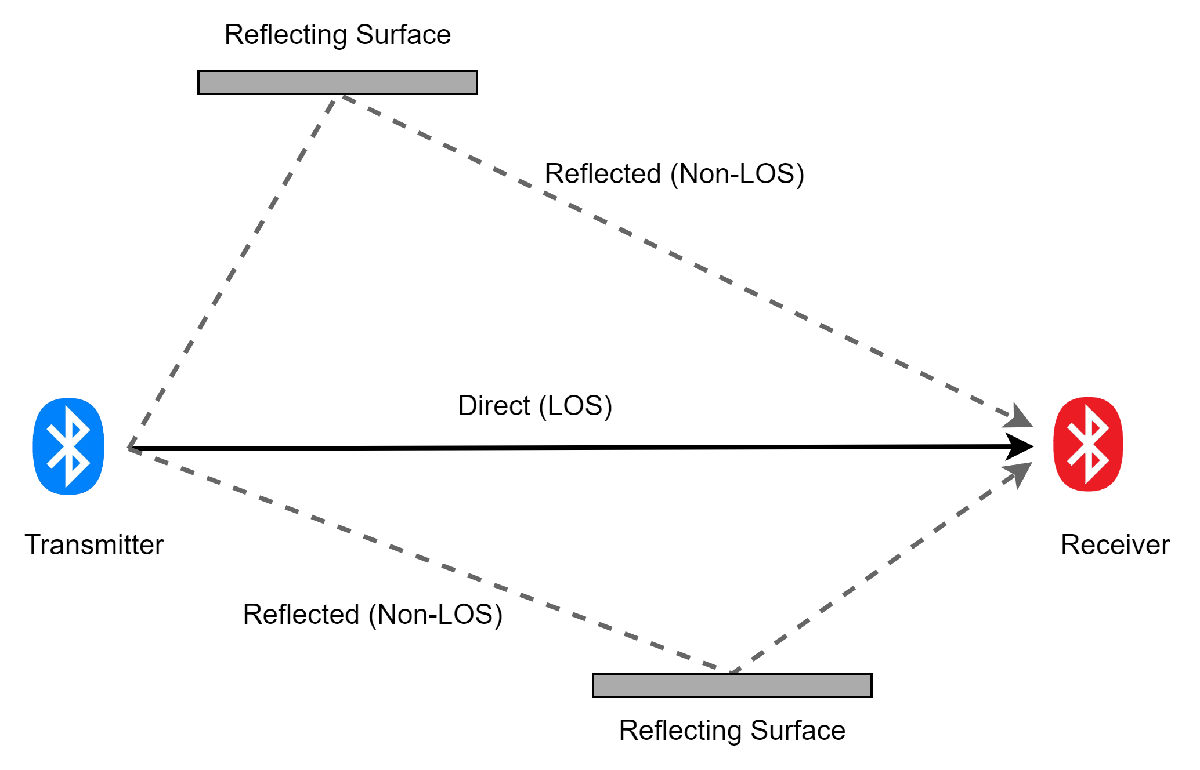The reflected paths non-line of sight (NLOS) result in the arrival of delayed versions of the signal at the receiver. In addition, the radio signal undergoes scattering on a local scale for each major path. Such local scattering typically results from reflections of objects near the receiver. These irresolvable components combine at the receiver and cause a phenomenon known as multipath fading. Due to this phenomenon, each major path behaves as a discrete fading path. Typically, the fading process is characterized by a Rayleigh distribution for an NLOS path and a Rician distribution for a LOS path.

#### Raytracing Channel Model

This channel model uses raytracing in an indoor environment between one transmitter site and one receiver site in a 3-D conference room. The channel model uses computed rays to construct a deterministic channel model specific to the Bluetooth LE Tx-Rx communication link. The same raytracing methods can be applied to build channel models for indoor and outdoor environments.

For more information about how to implement a raytracing channel model in an outdoor environment, see Urban Link and Coverage Analysis Using Ray Tracing.

### End-to-End Simulation Workflow

This figure shows the workflow of this end-to-end simulation. After you add the RF impairments, the Bluetooth LE waveforms are distorted with the fading channel model and AWGN. The noisy waveform is then received at the practical receiver.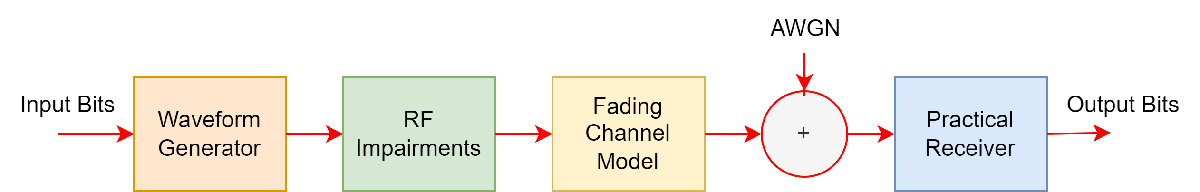This figure shows the operational workflow of the practical receiver.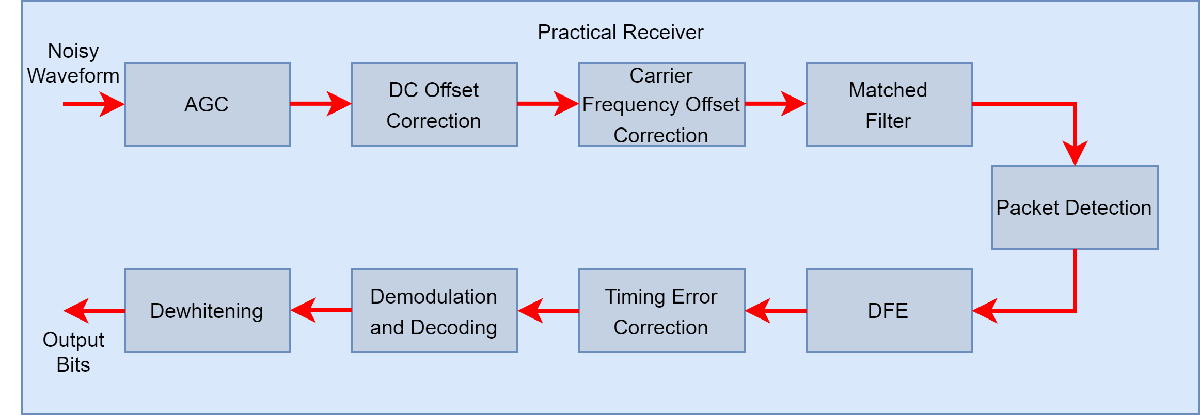The practical receiver receives the noisy waveforms as input and performs these operations.

1. Automatic gain control (AGC)

2. DC removal

3. Carrier frequency offset correction

4. Matched filtering

5. Packet detection

6. Decision feedback equalization (DFE)

7. Timing error correction

8. Demodulation and decoding

9. De-whitening

### Simulations

#### Configuration

Specify the noise power spectral density (Eb/No), samples per symbol, data length, and PHY transmission modes.

```EbNo = 2:4:24; % Eb/No in dB sps = 4; % Samples per symbol, must be greater than 1 dataLength =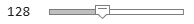128; % Data length in bytes, includes a header, payload and cycle redundancy check (CRC) simMode = {"LE1M","LE2M","LE500K","LE125K"}; % PHY transmission modes```

Specify the type of fading channel model as `"AWGN"`, `"Rician Channel"`, `"Rayleigh Channel"`, or `"Raytracing Channel"`.

`channelModel =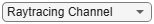"Rician Channel" ;`

Enable or disable the DFE by checking the `enableEqualizer` flag. Enabling DFE will mitigate the effects of the fading channel model on the Bluetooth LE transmit waveforms.

`enableEqualizer =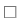false;`

These parameters control the number of packets tested at each Eb/No point.

• `maxNumErrors: `This parameter specifies the maximum number of bit errors simulated at each Eb/No point. When the number of bit errors reaches this value, the simulation at this Eb/No is complete.

• `maxNumPackets:` This parameter specifies the maximum number of packets simulated at each Eb/No point. When the number of packets reaches this value, the simulation at this Eb/No is complete.

Specify the `maxNumErrors` and `maxNumPackets` values. For the purpose of this example, specify small values for `maxNumErrors` and `maxNumPackets`. For statistically meaningful results, you can simulate the example with higher `maxNumErrors` and `maxNumPackets` values.

```maxNumErrors = 10; maxNumPackets = 100;```

Get the number of PHY modes for simulation.

`numMode = numel(simMode);`

Pre-allocate the space to store the BER and PER results.

`[ber,per] = deal(zeros(numMode,length(EbNo)));`

If you specify the channel model as "`Raytracing Channel`", pre-allocate the space to store data for the site viewer.

```if channelModel=="Raytracing Channel" visualVar = cell(numMode,length(EbNo)); end```

Specify the number of bits per byte.

`bitsPerByte = 8; `

For each PHY mode, set the signal-to-noise ratio (SNR). For coded PHYs (LE500K and LE125K), the SNR calculation includes the 1/2 code rate forward error correction (FEC) encoder.

```for countMode = 1:numMode phyMode = simMode{countMode}; if any(phyMode==["LE1M","LE2M"]) snrVec = EbNo - 10*log10(sps); else codeRate = 1/2; snrVec = EbNo + 10*log10(codeRate) - 10*log10(sps); end```

Sampling frequency of the generated waveform

` sampleRate = sps*(1+(phyMode=="LE2M"))*1e6;`

#### Simulate for Each Eb/No Point

This example also demonstrates how a `parfor` loop can be used instead of the `for` loop when simulating each Eb/No point to speed up a simulation. parfor, as part of the Parallel Computing Toolbox™, executes processing for each Eb/No point in parallel to reduce the total simulation time.

To enable the use of parallel computing for increased simulation speed, use parfor by commenting out the `for` statement. To use `parfor`, you must install Parallel Computing Toolbox. If Parallel Computing Toolbox is not installed, `parfor` will default to the `for` statement.

``` % parfor countSnr = 1:length(snrVec) % Use "parfor" to speed up the simulation for countSnr = 1:length(snrVec) % Use "for" to debug the simulation```

To ensure that each iteration uses a repeatable set of random numbers, set a random substream index for each iteration.

``` stream = RandStream("combRecursive",Seed=100); stream.Substream = countSnr; RandStream.setGlobalStream(stream);```

Create an instance of error rate.

``` errorRate = comm.ErrorRate(Samples="Custom", ... CustomSamples=1:(dataLength*bitsPerByte-1));```

To configure the RF impairments, use the `helperBLEImpairmentsInit` helper object.

` initImp = helperBLEImpairmentsInit(phyMode,sps);`

Initialize and update the fading channel model.

``` channelInit = cell(1,1); if channelModel~="AWGN" channelInit = helperBLEChannelInit(phyMode,sps,channelModel); end ```

Configure the parameters of the practical receiver.

``` rxCfg = struct(Mode=phyMode,SamplesPerSymbol=sps,ChannelIndex=37, ... DFPacketType="Disabled"); rxCfg.CoarseFreqCompensator = comm.CoarseFrequencyCompensator(Modulation="OQPSK", ... SampleRate=sampleRate, ... SamplesPerSymbol=2*sps, ... FrequencyResolution=30); rxCfg.PreambleDetector = comm.PreambleDetector(Detections="First");```

Initialize the parameters for error computation.

``` [numErrs,perCnt] = deal(0); numPkt = 1;```

Reset the channel for different interations of SNR.

``` if channelModel~="AWGN" reset(channelInit.fadingChan); end```

Configure and generate the Bluetooth LE waveform.

``` while numErrs <= maxNumErrors && numPkt <= maxNumPackets txBits = randi([0 1],dataLength*bitsPerByte,1,"int8"); % Data bits generation channelIndex = randi([0 39],1,1); % Random channel index value for each packet if channelIndex <= 36 % Random access address for data channels. This access % address value meets the requirements specified in Section % 2.1.2, Part-B, Vol-6 of Bluetooth specification. accessAddress = [1 0 0 0 1 1 1 0 1 1 0 0 1 ... 0 0 1 1 0 1 1 1 1 1 0 1 1 0 1 0 1 1 0]'; else % Default access address for periodic advertising channels accessAddress = [0 1 1 0 1 0 1 1 0 1 1 1 1 1 0 1 1 0 0 ... 1 0 0 0 1 0 1 1 1 0 0 0 1]'; end txWaveform = bleWaveformGenerator(txBits,Mode=phyMode, ... SamplesPerSymbol=sps, ... ChannelIndex=channelIndex, ... AccessAddress=accessAddress);```

Configure and add the RF impairment parameters to the generated Bluetooth LE waveform.

``` initImp.pfo.FrequencyOffset = randsrc(1,1,-50e3:10:50e3); % Frequency offset in Hz, range is [-150000,+150000] initImp.pfo.PhaseOffset = randsrc(1,1,-10:5:10); % phase offset in degrees initoff = 0.15*sps; % static timing offset stepsize = 20*1e-6; % Timing drift in ppm, max range is +/- 50 ppm initImp.vdelay = (initoff:stepsize:initoff+stepsize*(length(txWaveform)-1))'; % Variable timing offset initImp.dc = 20; % Percentage with regard to maximum amplitude value txImpairedWfm = helperBLEImpairmentsAddition(txWaveform,initImp);```

Pass the impaired waveform through the fading channel.

``` if (channelModel=="AWGN") txChanWfm = txImpairedWfm; else % Fading channel filter delay chanDelay = info(channelInit.fadingChan).ChannelFilterDelay; % Pass through the fading channel model txChanWfm = channelInit.fadingChan([txImpairedWfm; zeros(chanDelay,1)]); txChanWfm = txChanWfm(chanDelay+1:end,1); if channelModel=="Raytracing Channel" visualVar{countMode,countSnr} = channelInit.VisualVar; end end```

` rxWaveform = awgn(txChanWfm,snrVec(countSnr),"measured"); `

Recover data bits from the noisy waveform using the practical receiver.

``` rxCfg.ChannelIndex = channelIndex; rxCfg.AccessAddress = accessAddress; rxCfg.EqualizerFlag = enableEqualizer; [rxBits,recAccessAddress] = helperBLEPracticalReceiver(rxWaveform,rxCfg);```

Determine the BER and PER by comparing transmitted and received bits.

``` if(length(txBits) == length(rxBits)) errors = errorRate(txBits,rxBits); % Outputs the accumulated errors ber(countMode,countSnr) = errors(1);% Accumulated BER currentErrors = errors(2)-numErrs; % Number of errors in current packet if(currentErrors) % Check if the current packet is in error or not perCnt = perCnt + 1; % Increment the PER count end numErrs = errors(2); % Accumulated errors numPkt = numPkt + 1; % Increment the packet number end end per(countMode,countSnr) = perCnt/(numPkt-1); % Display message for the particular value of SNR disp(join(["Mode ",phyMode,",", ... " simulating for ",channelModel," model,", ... " Eb/No = ",num2str(EbNo(countSnr)),"dB,", ... " data length = ",num2str(dataLength),"bytes,", ... " BER: ",num2str(ber(countMode,countSnr)),",", ... " PER: ",num2str(per(countMode,countSnr))],"")); end end```
```Mode LE1M, simulating for Rician Channel model, Eb/No = 2dB, data length = 128bytes, BER: 0.20723, PER: 1 Mode LE1M, simulating for Rician Channel model, Eb/No = 6dB, data length = 128bytes, BER: 0.074291, PER: 1 Mode LE1M, simulating for Rician Channel model, Eb/No = 10dB, data length = 128bytes, BER: 0.01564, PER: 1 Mode LE1M, simulating for Rician Channel model, Eb/No = 14dB, data length = 128bytes, BER: 0.0068426, PER: 0.5 Mode LE1M, simulating for Rician Channel model, Eb/No = 18dB, data length = 128bytes, BER: 0.00034213, PER: 0.075 Mode LE1M, simulating for Rician Channel model, Eb/No = 22dB, data length = 128bytes, BER: 0, PER: 0 Mode LE2M, simulating for Rician Channel model, Eb/No = 2dB, data length = 128bytes, BER: 0.13294, PER: 1 Mode LE2M, simulating for Rician Channel model, Eb/No = 6dB, data length = 128bytes, BER: 0.056696, PER: 1 Mode LE2M, simulating for Rician Channel model, Eb/No = 10dB, data length = 128bytes, BER: 0.013685, PER: 1 Mode LE2M, simulating for Rician Channel model, Eb/No = 14dB, data length = 128bytes, BER: 0.00041894, PER: 0.17857 Mode LE2M, simulating for Rician Channel model, Eb/No = 18dB, data length = 128bytes, BER: 1.955e-05, PER: 0.01 Mode LE2M, simulating for Rician Channel model, Eb/No = 22dB, data length = 128bytes, BER: 0, PER: 0 Mode LE500K, simulating for Rician Channel model, Eb/No = 2dB, data length = 128bytes, BER: 0.3001, PER: 1 Mode LE500K, simulating for Rician Channel model, Eb/No = 6dB, data length = 128bytes, BER: 0.072336, PER: 1 Mode LE500K, simulating for Rician Channel model, Eb/No = 10dB, data length = 128bytes, BER: 0.016618, PER: 1 Mode LE500K, simulating for Rician Channel model, Eb/No = 14dB, data length = 128bytes, BER: 0.00046548, PER: 0.071429 Mode LE500K, simulating for Rician Channel model, Eb/No = 18dB, data length = 128bytes, BER: 0, PER: 0 Mode LE500K, simulating for Rician Channel model, Eb/No = 22dB, data length = 128bytes, BER: 0, PER: 0 Mode LE125K, simulating for Rician Channel model, Eb/No = 2dB, data length = 128bytes, BER: 0.12414, PER: 1 Mode LE125K, simulating for Rician Channel model, Eb/No = 6dB, data length = 128bytes, BER: 0.0013848, PER: 0.16667 Mode LE125K, simulating for Rician Channel model, Eb/No = 10dB, data length = 128bytes, BER: 0, PER: 0 Mode LE125K, simulating for Rician Channel model, Eb/No = 14dB, data length = 128bytes, BER: 0, PER: 0 Mode LE125K, simulating for Rician Channel model, Eb/No = 18dB, data length = 128bytes, BER: 0, PER: 0 Mode LE125K, simulating for Rician Channel model, Eb/No = 22dB, data length = 128bytes, BER: 0, PER: 0 ```

### Results and Visualizations

Specify the marker, color, and space for the legend variable.

```marker = "ox*s"; color = "bmgr"; legendVar = {zeros(numMode,1)};```

Plot the BER and the PER curves for each PHY modes.

```for countMode = 1:numMode subplot(2,1,1),semilogy(EbNo,ber(countMode,:).',"-"+marker{1}(countMode)+color{1}(countMode)); hold on; legendVar(countMode) = simMode(countMode); subplot(2,1,2),semilogy(EbNo,per(countMode,:).',"-"+marker{1}(countMode)+color{1}(countMode)); hold on; legendVar(countMode) = simMode(countMode); end subplot(2,1,1), grid on; xlabel("Eb/No (dB)"); ylabel("BER"); legend(legendVar); title(join(["BER of Bluetooth LE communication in ",channelModel],"")); subplot(2,1,2), grid on; xlabel("Eb/No (dB)"); ylabel("PER"); legend(legendVar); title(join(["PER of Bluetooth LE communication in ",channelModel],""));```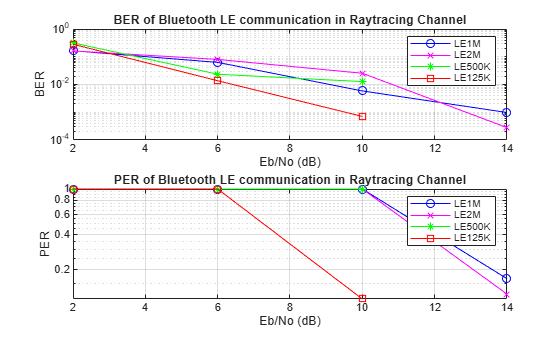Visualize Raytracing Model

This image shows a conference room environment to simulate Bluetooth LE communication in the presence of the raytracing channel model.

```if (channelModel=="Raytracing Channel") visualVar = visualVar{end,end}; % Show conference room viewer = siteviewer(SceneModel=visualVar.MapFileName); % Set the icons for transmit site and receiver site show(visualVar.TxSite,Icon="bleTxIcon.png"); show(visualVar.RxSite,Icon="bleRxIcon.png"); % Plot the rays in the site viewer plot(visualVar.Rays,Type="power",ColorLimits=[-30 0]); end```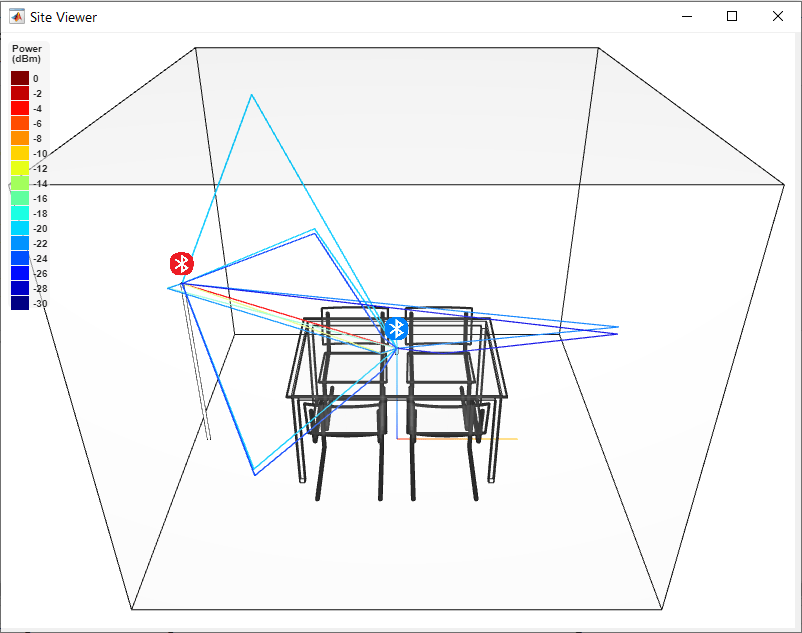The preceding scenario consists of a 3-D indoor conference room, where the blue Bluetooth icon indicates the transmit site and the red Bluetooth icon indicates the receiver site. The rays in the scenario contain LOS components and NLOS components. Each ray reflects its color based on its path loss value. All the rays converge at the receiver site after zero, one, or two interactions (such as reflection and scattering) with a reflecting surface.

### Further Exploration

You can further explore this example by increasing the `maxNumErrors` and `maxNumPackets` parameters. These BER and PER results are obtained by using this configuration.

• `dataLength `— 128 bytes

• `maxNumErrors `— 1e3

• `maxNumPackets `— 1e4

• `phyMode `— LE1M

• `channelModel `— Rician, Rayleigh and Raytracing channel model

To compensate for the fading channel effects, channel equalization is added to the simulation.

The figure shows the BER and PER obtained for three scenarios:

• AWGN: Simulation does not contain any impairments.

• Fading Channel with Equalizer: Simulation contains RF impairments and fading channel conditions with equalization.

LE1M in Rician Channel ModelLE1M in Rayleigh Channel ModelLE1M in Raytracing Channel ModelThe reference Eb/No values generated based on the Bluetooth LE specification include a margin for RF impairments that are not modeled in this simulation. As a result, these simulation results outperform the standard reference results. If you modify this example to include additional impairments such as frequency drift and interference, the BER and PER values increase with respect to the reference Eb/No values specified in .

### Appendix

The example uses these helpers:

### Selected Bibliography

 “Bluetooth® Technology Website – The Official Website for the Bluetooth Wireless Technology. Get up to Date Specifications, News, and Development Info.” Accessed January 4, 2023. https://www.bluetooth.com/.

 “Core Specification – Bluetooth® Technology Website.” Accessed January 4, 2023. https://www.bluetooth.com/specifications/specs/core-specification-5-3/.

 “M.1225: Guidelines for Evaluation of Radio Transmission Technologies for IMT-2000.” Accessed January 4, 2023.https://www.itu.int/rec/R-REC-M.1225/en.